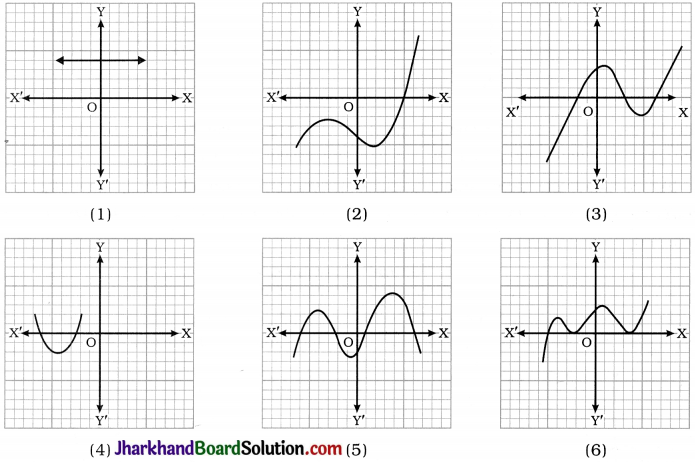# JAC Class 10 Maths Solutions Chapter 2 Polynomials Ex 2.1

Jharkhand Board JAC Class 10 Maths Solutions Chapter 2 Polynomials Ex 2.1 Textbook Exercise Questions and Answers.

## JAC Board Class 10 Maths Solutions Chapter 2 Polynomials Exercise 2.1

Question 1.Solution:
1. The number of zeroes is 0 as the graph being parallel to the x-axis does not intersect it.
2. The number of zeroes is 1 as the graph intersects the x-axis at one point only.
3. The number of zeroes is 3 as the graph intersects the x-axis at three points.
4. The number of zeroes is 2 as the graph intersects the x-axis at two points.
5. The number of zeroes is 4 as the graph intersects the x-axis at four points.
6. The number of zeroes is 3 as the graph intersects the x-axis at three points.